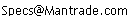Daily Speculations The Web Site of Victor Niederhoffer & Laurel Kenner Dedicated to the scientific method, free markets, deflating ballyhoo, creating value, and laughter;  a forum for us to use our meager abilities to make the world of specinvestments a better place.

Write to us at:(address is not clickable)

9/14/04
Victor Niederhoffer on Reducing Uncertainty

The information content of a message is the reduction of uncertainty it causes, measured in bits. Knowledge that an event with a probability of 1/4 has happened yields 2 bits of information. Knowledge of an event with a probability of 1/2 gives 1 bit of information. If p is the probability of an event, then log (1/p) is the information content.

The concept can be readily generalized and is done so quite nicely by the late econometrics pioneer Henri Theil in his highly recommended but unfortunately out-of-print 1967 book Economics and Information Theory. Theil wrote that the information content of the message = uncertainty before the event (ex ante) less the uncertainty after the event (ex post). If x1 is the probability the event will occur before and x2 is the probability the event will occur after, the information content is log (1/x1) less log (1/x2 ) = log (x2/x1).

For example, if beforehand you gave an event probability of 1/4 and after, 1/2, then 1 bit of information was gained. Since only 2 bits of information were possible to be gained, the percentage of the total information gained was 0.50.

The concept has myriad applications in markets. One thinks of the case where earnings have been forecast either to increase or decrease. The probability of an actual earnings increase might be 0.70 ex ante. When it's actually predicted to increase, the probability might be 0.80. That's a reduction of uncertainty of 0.19 bits out of a total of 0.51, or 38%. (The log of 1/0.7 is 0.51 and the log of 1/0.8 is 0.32).

Other useful areas are to determine amount of information given by an announcement such as employment or consumer prices or an election winner, and also to measure the accuracy of predictions.

Looking at weekly bonds and stocks over the past six months, I come up with the following 2x2 table:

 Current Week's Change in Bonds Actual Change Next Week in Stocks Increase Decrease Increase 6 4 10 Decrease 6 8 14 Marginal 12 12 24

The ex ante probability of an increase in stocks is 0.50. When bonds were up the previous week, the probability went to 0.60. Thus, 0.27 bits of information out of 1 bit, or 27% of the maximum possible reduction, was obtained when the previous bonds were up.

The ex ante probability of an decrease in stocks was 0.50. When a decrease occurred, the probability of a decrease in stocks went up to 8/14, or 0.56. Thus, 0.17, or 17% of the maximum possible reduction of uncertainty, was obtained.

It will be interesting to see which of such things as earnings increase or earnings decrease, upgrades in ratings or downgrades, increases or decreases in GDP growth, up or down revisions et al. will show more information content.